Refer to our Texas Go Math Grade 1 Answer Key Pdf to score good marks in the exams. Test yourself by practicing the problems from Texas Go Math Grade 1 Lesson 7.2 Answer Key Think Addition to Subtract.

Essential Question
How can you use an addition fact to find the answer to a subtraction fact?
Explanation:
The basic facts of addition are those equations in which two single-digit numbers are combined by addition to give a sum Hence they range from 0+0=0 to 9+9=18. For each basic addition fact there is a related basic subtraction fact, for example, 18-9=9.

Explore
Use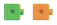to model the problem.
Drawto show your work.

What is 12 – 5 ?

5 + ___ = 1212 – 5 = ___For The Teacher

• Read the following problems. Joey had 5 cubes. Sarah gave him more cubes Now Joey has 12 cubes. How many cubes did Sarah give him? Children use the top workspace to solve. Then have children solve this problem: Joey had 12 cubes. He gave Sarah 5 cubes. How many cubes does Joey have flow?

Math Talk
Mathematical Processes
Explain how 5 + 7 = 12 can help you find 12 – 5.
Explanation:
5 + 7 = 12
12 – 5 = 7
This type of equations are called subtraction facts.

Model and Draw

What is 9 – 4?Explanation:Share and Show

Use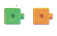to add and to subtract.

Question 1.
What is 8 – 6?Explanation:
used an addition fact to find the answer to a subtraction fact

Question 2.
What is 8 – 4?Explanation:
used an addition fact to find the answer to a subtraction fact

Question 3.
‘What is 10 – 4?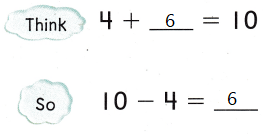Explanation:
used an addition fact to find the answer to a subtraction fact

Question 4.
What is 12 – 6?Explanation:
used an addition fact to find the answer to a subtraction fact

Problem Solving

Use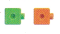to add and to subtract.

Question 5.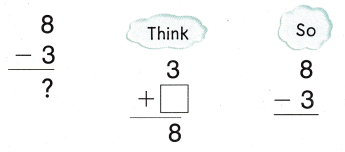Explanation:
used an addition fact to find the answer to a subtraction fact

Question 6.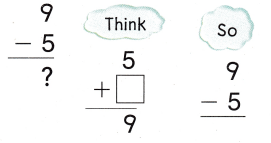Explanation:
used an addition fact to find the answer to a subtraction fact

Question 7.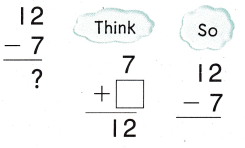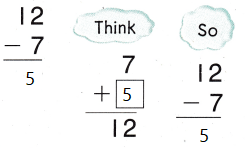Explanation:
used an addition fact to find the answer to a subtraction fact

Solve.

Question 8.
H.O.T. Carol can use an addition sentence to write a subtraction sentence. Write a subtraction sentence she can solve using 6 + 8 = 14.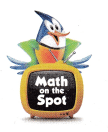6 + 8 = 14
14 – 8 = 6
Explanation:a subtraction sentence she can solve using 6 + 8 = 14.

Question 9.
H.O.T. Multi-Step Write two addition sentences Carol can use to help her solve 13 — 9.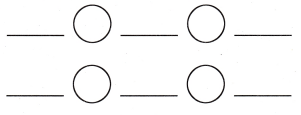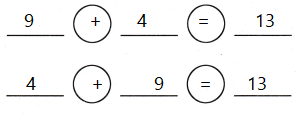Explanation:
Carol  used this two addition sentences
9 + 4 = 13
4 + 9 = 13 to solve the subtraction sentence
13 – 9 = 4

Question 10.
Analyze Which addition sentence helps you solve the problem? Fritz buys 12 balloons for the party. 5 balloons are red. The rest are green. How many balloons are green?(A) 2 + 3 = 5
(B) 5 + 7 = 12
(C) 6 + 6 = 12
Explanation:
5 + 7 = 12 helps you solve the problem.
Fritz buys 12 balloons for the party. 5 balloons are red. The rest are green.
12 – 5 = 7
7  balloons are green

Question 11.
Which addition sentence helps you solve the problem?
Ben had 9 toy cars. He gave away 3 toy cars. How many toy cars does Ben have now?
(A) 3 + 6 = 9
(B) 8 + 1 = 9
(C) 9 + 3 = 12Explanation:
Ben had 9 toy cars. He gave away 3 toy cars.
9 – 3 = 6
6 toy cars that Ben have now

Question 12.
Which subtraction sentence can you solve using 7 + 6 = 13?
(A) 7 – 6 = ____
(B) 13 – 6 = ___
(C) 13 – 8 = ____
Explanation:
13 – 6 = 7 is the subtraction sentence
which is derived from the addition fact.

Question 3.
Texas Test Prep Which addition sentence helps you solve 10 — 7?
(A) 3 + 4 = 7
(B) 5 + 5 = 10
(C) 7 + 3 = 10
Explanation:
7 + 3 = 10 is the addition sentence which is derived
from the subtraction sentence
10 – 7 =3

Take Home Activity
• Write 5 + 4 = __, and ask your child to write the sum Have him or her explain how to use 5 + 4 = 9, to solve, __ – 4 = 5, and then write the answer.
Explanation:
5 + 4 = 9
9 – 4 = 5
Are the facts of addition and subtraction.

### Texas Go Math Grade 1 Lesson 7.2 Homework and Practice Answer Key

Question 1.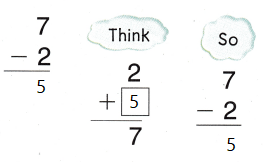Explanation:
used an addition fact to find the answer to a subtraction fact

Question 2.Explanation:
used an addition fact to find the answer to a subtraction fact

Question 3.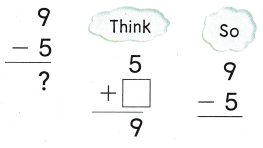Explanation:
used an addition fact to find the answer to a subtraction fact

Question 4.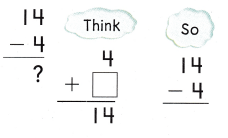Explanation:
used an addition fact to find the answer to a subtraction fact

Problem Solving

Question 5.
Multi-Step Write two addition sentences Claire can use to help solve 14 — 5.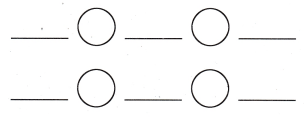Explanation:
The above 2 equations are addition sentences
derived from subtraction sentences.

Texas Test Prep

Lesson Check

Question 6.
Which addition sentence helps you solve the problem?
There are 11 balls. 6 are footballs. The rest are soccer balls. How many balls are soccer balls?(A) 4 + 7 = 1
(B) 6 + 5 = 11
(C) 3 + 3 = 6
Explanation:
There are 11 balls. 6 are footballs.
11 – 6 = 5
The rest are soccer balls.
5 balls are soccer balls

Question 7.
Which addition sentence helps you solve the problem?
Bailey had 13 purses. She gave away 5 purses. How many purses does she have now?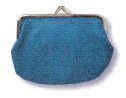(A) 5 + 6 = 11
(B) 9 + 5 = 14
(C) 5 + 8 = 13
Explanation:
Bailey had 13 purses. She gave away 5 purses.
13 – 5 = 8
8 purses that she have now

Question 8.
Which subtraction sentence can you solve using 8 + 6 = 14?
(A) 8 – 6 = 2
(B) 14’ – 6 = 8
(C) 14 – 7 = 7
Explanation:
14’ – 6 = 8 is the subtraction sentence
that can be used to solve by using 8 + 6 = 14

Question 9.
Which addition sentence can you use to solve 13 – 7?
(A) 7 + 6 = 13
(B) 7 + 4 = 11
(C) 5 + 8 = 13